# Telangana SSC Board (TS SSC) Question Paper 1 for Class 10th Maths 2016 In PDF

## Telangana 10th Annual Exam Question Papers 2016 Maths Paper 1 with Solutions – Free Download

Telangana SSC Class 10 Maths 2016 Question Paper 1 with solutions given at BYJU’S are provided by the subject experts. These Telangana SSC Question Paper 2016 Maths Paper 1 solutions are given by considering the marking scheme prescribed by the board. All such steps which hold specific marks are covered in the solutions of all TS SSC Class 10 Maths previous year papers at BYJU’S. Students can get SSC textbooks of Telangana board along with the syllabus, so that they get acquainted with the exam pattern. Students can also go through with the important concepts of Class 10 Maths at BYJU’S to improve their knowledge on fundamentals.

### Telangana Board SSC Class 10 Maths 2016 Question Paper 1 with Solutions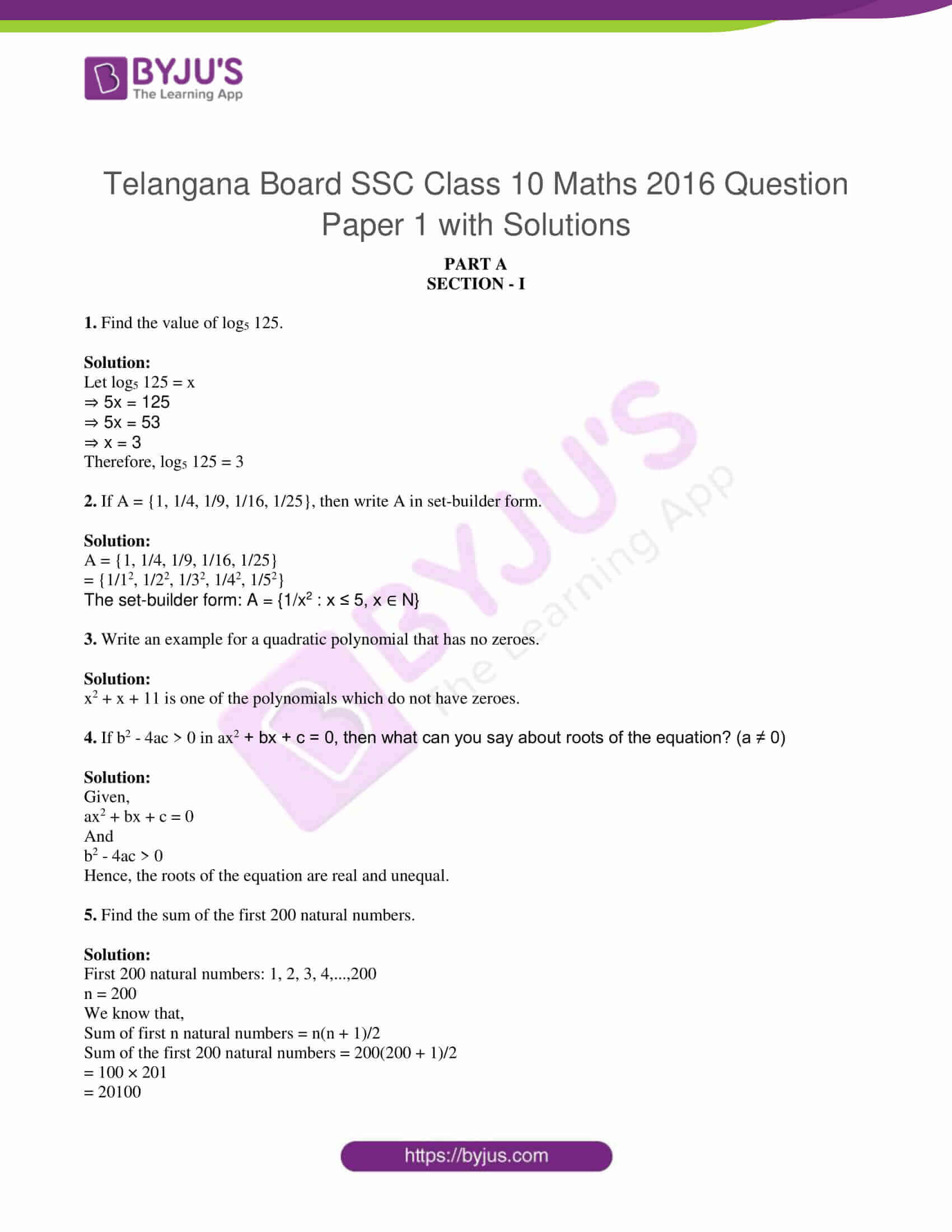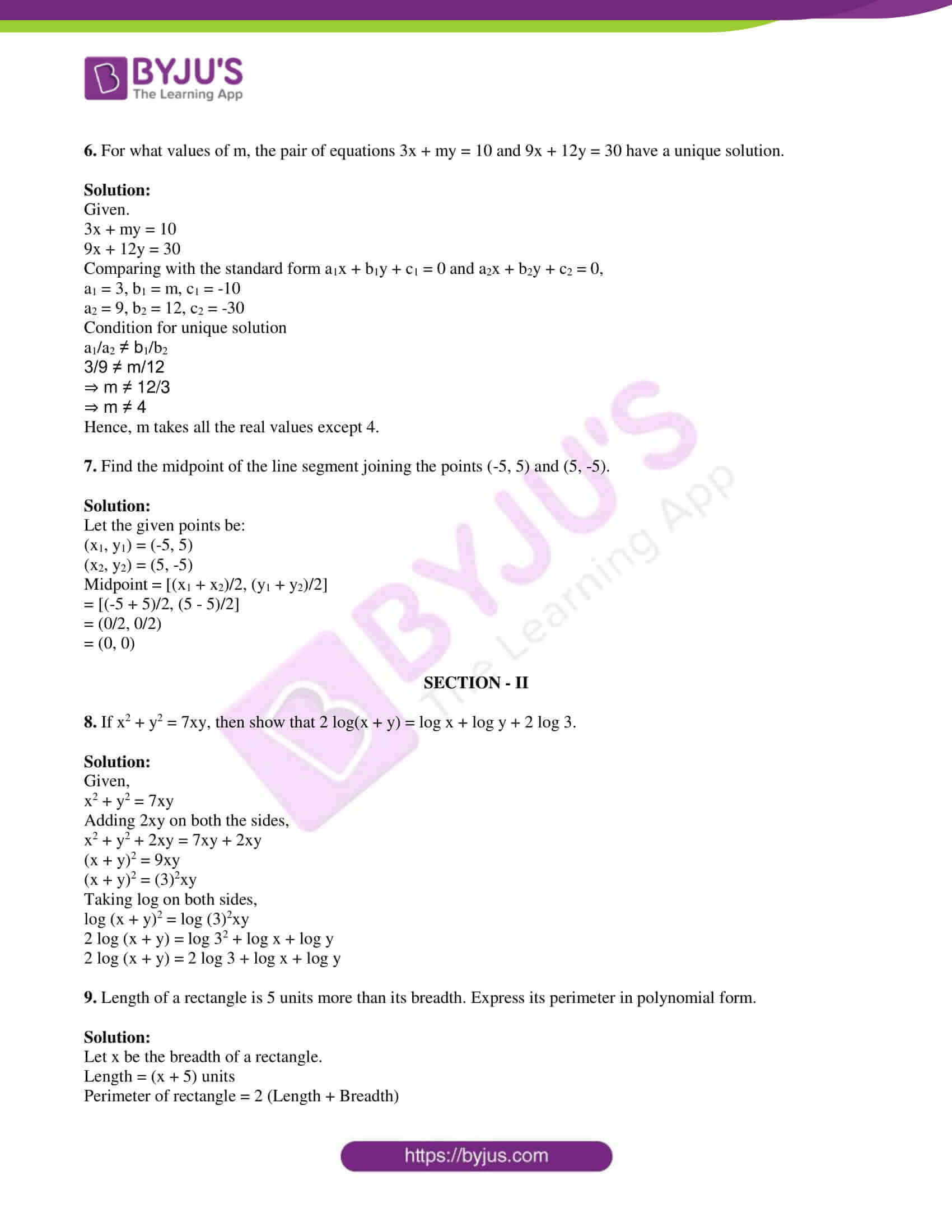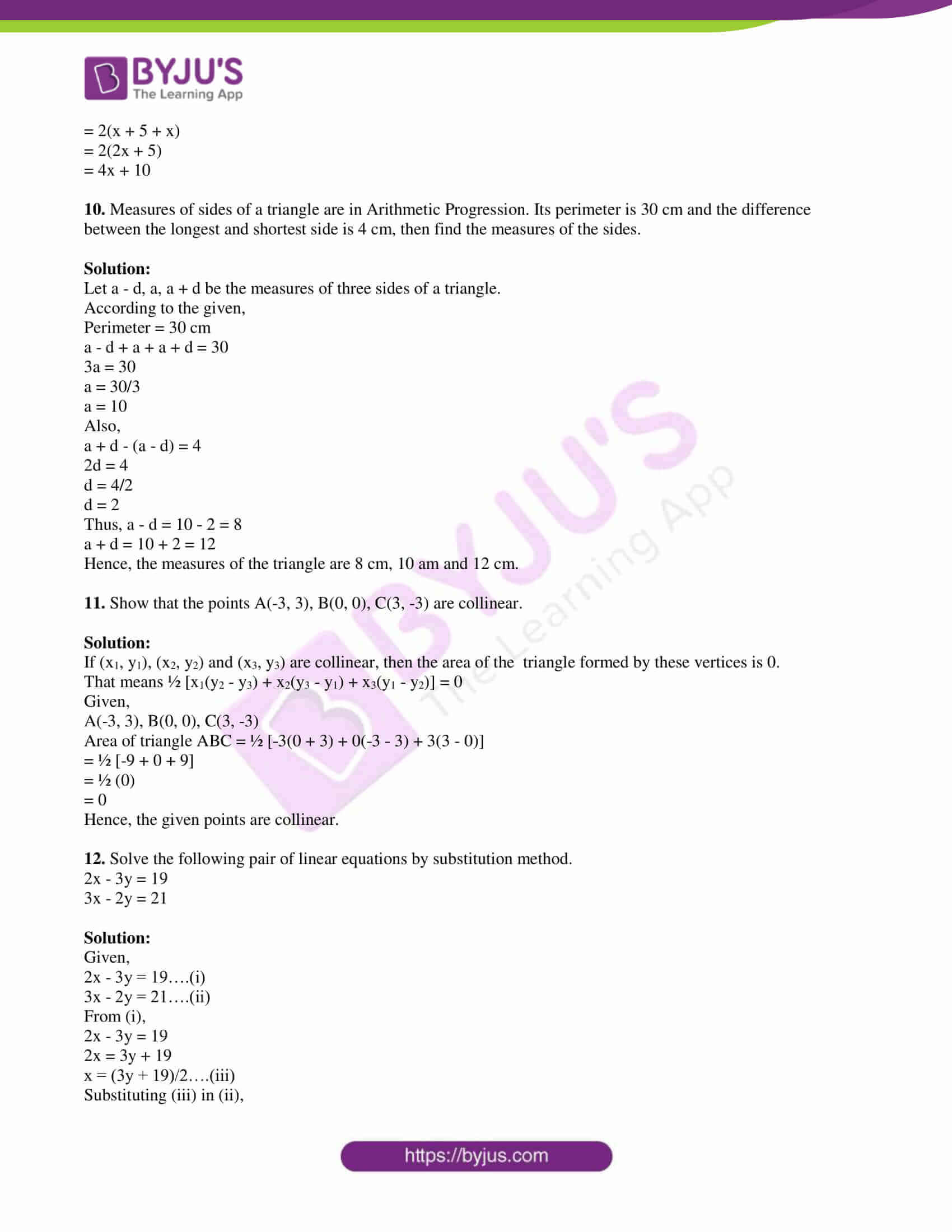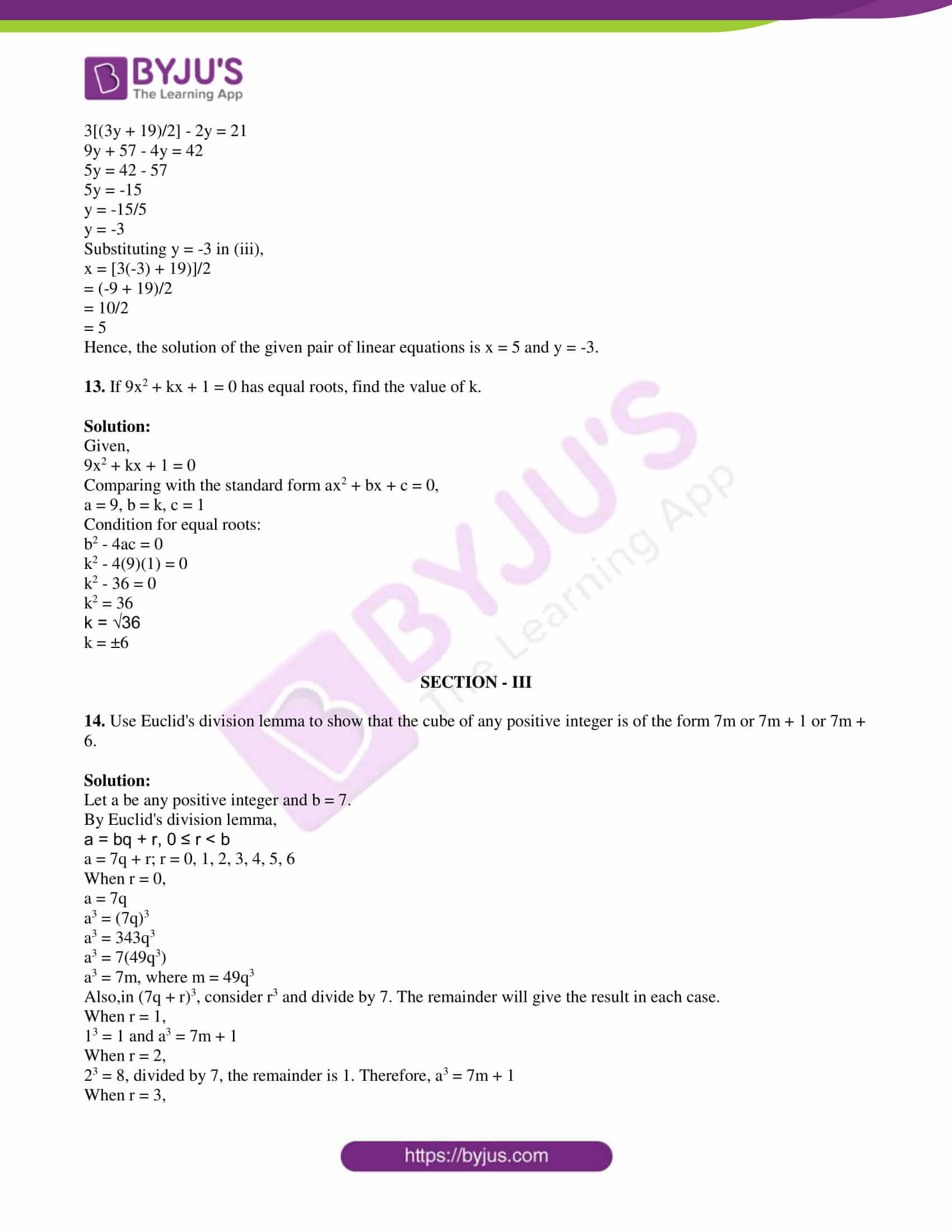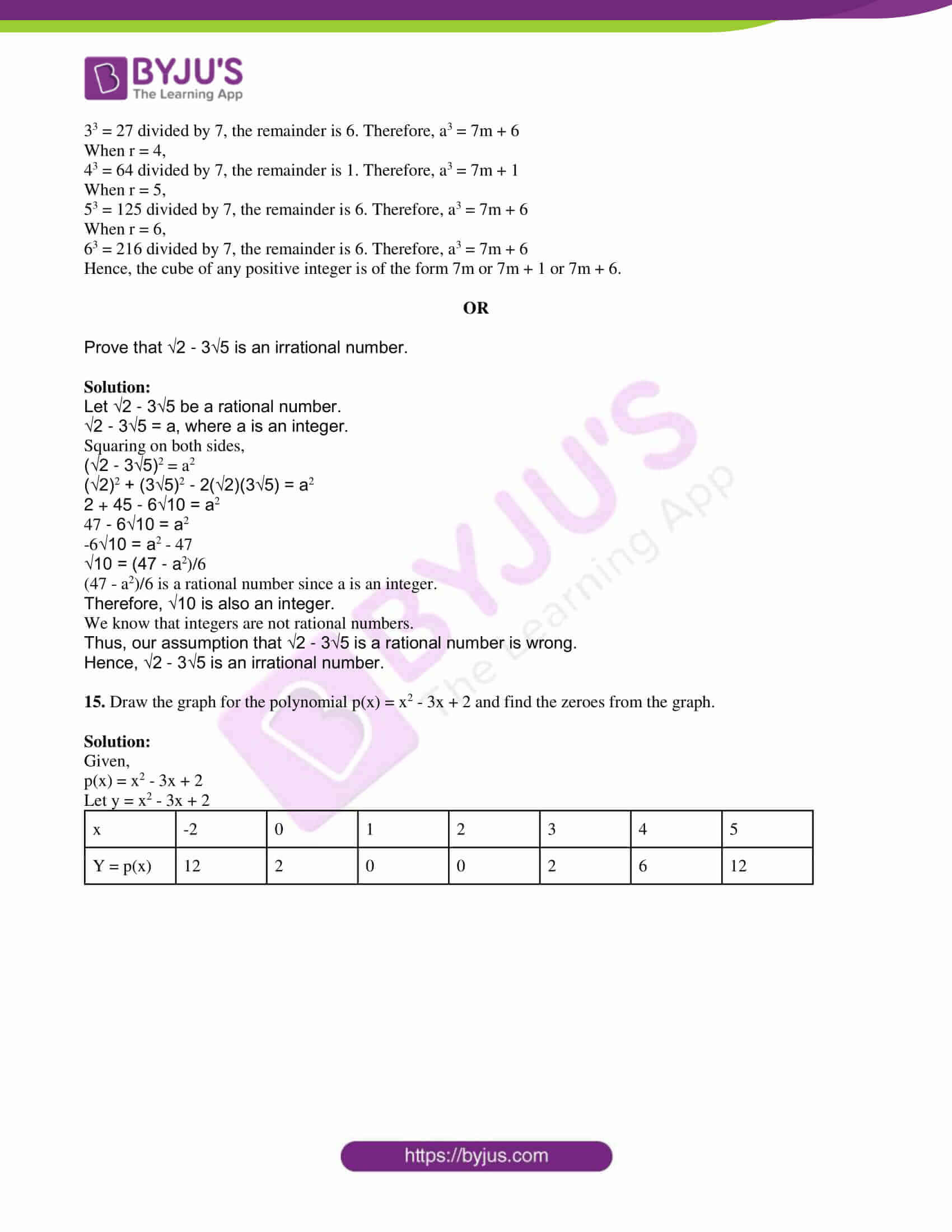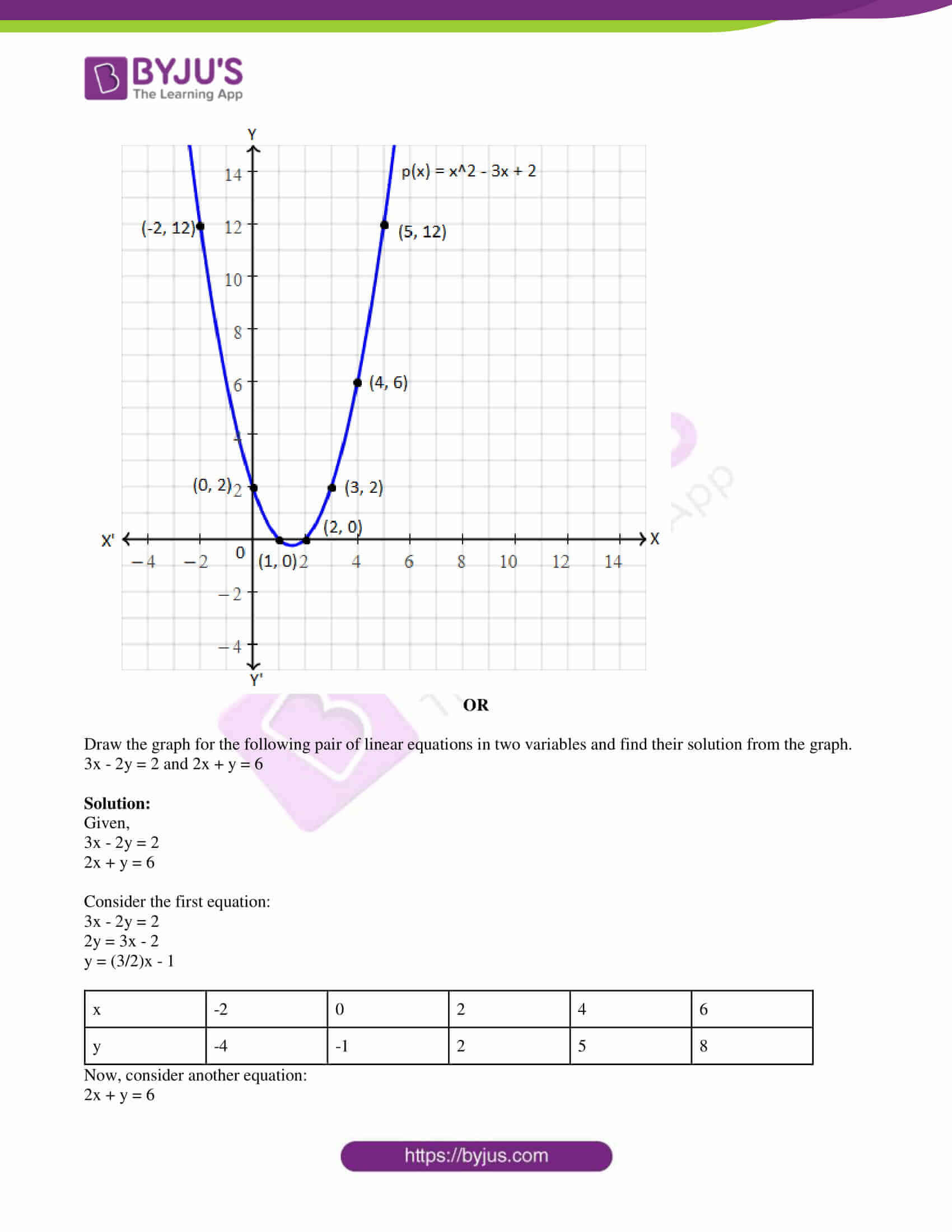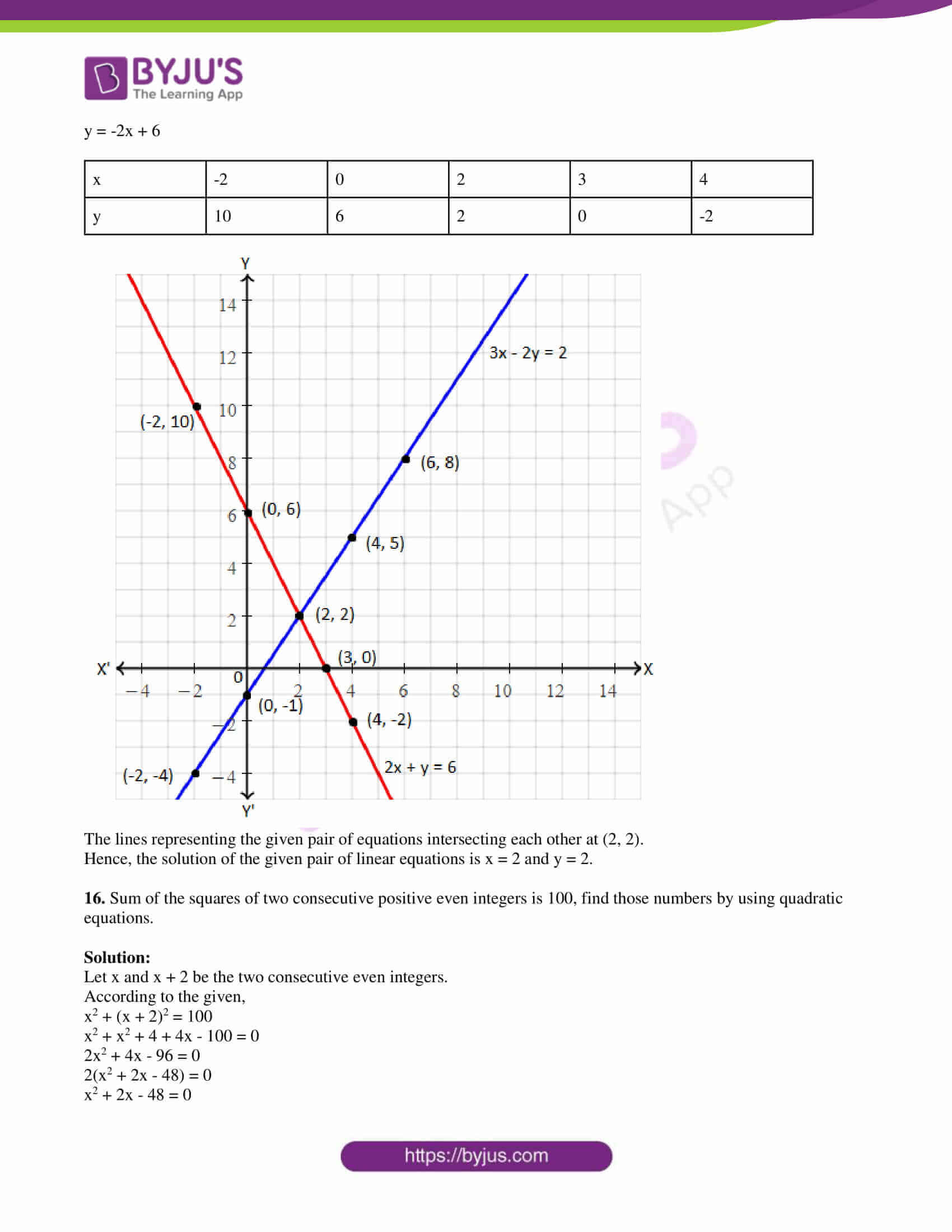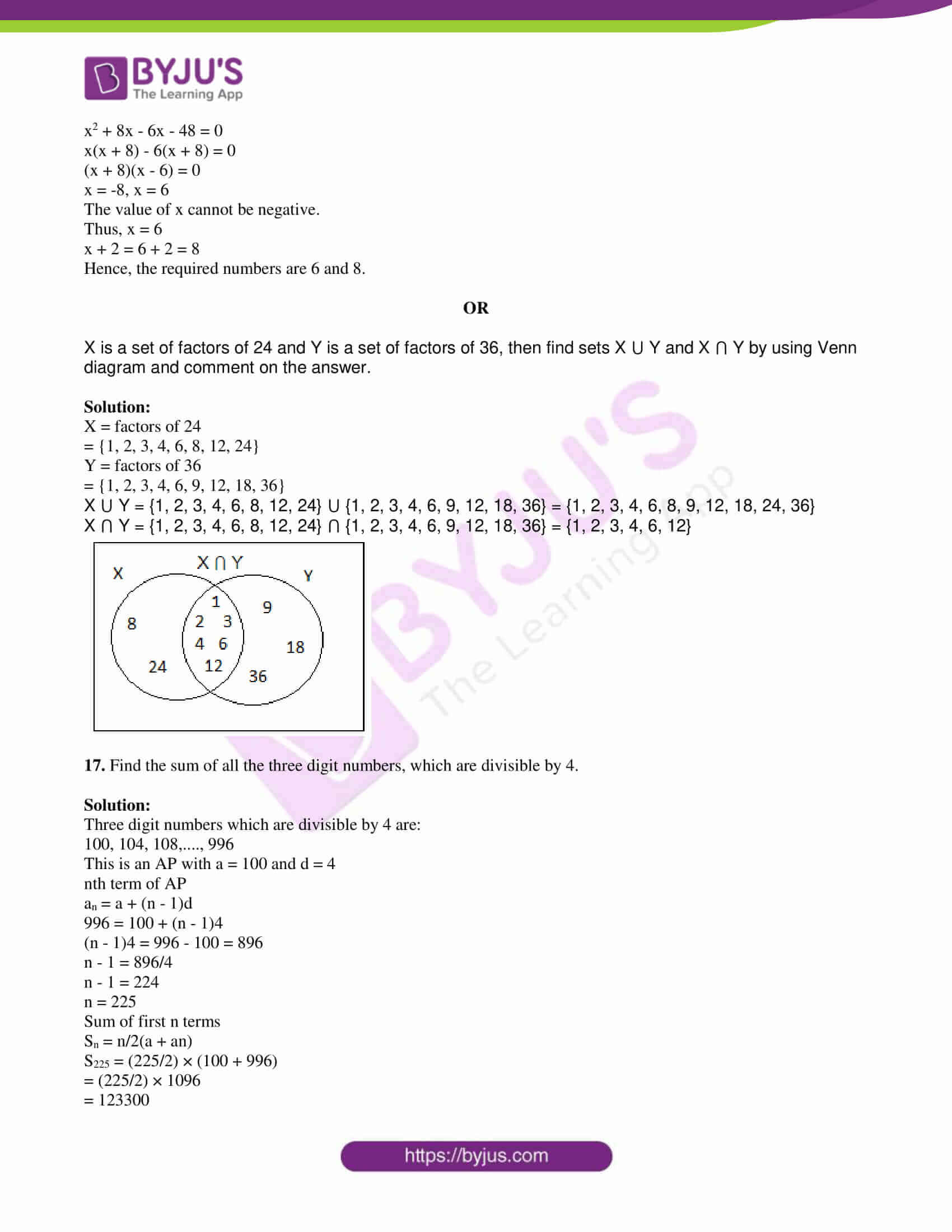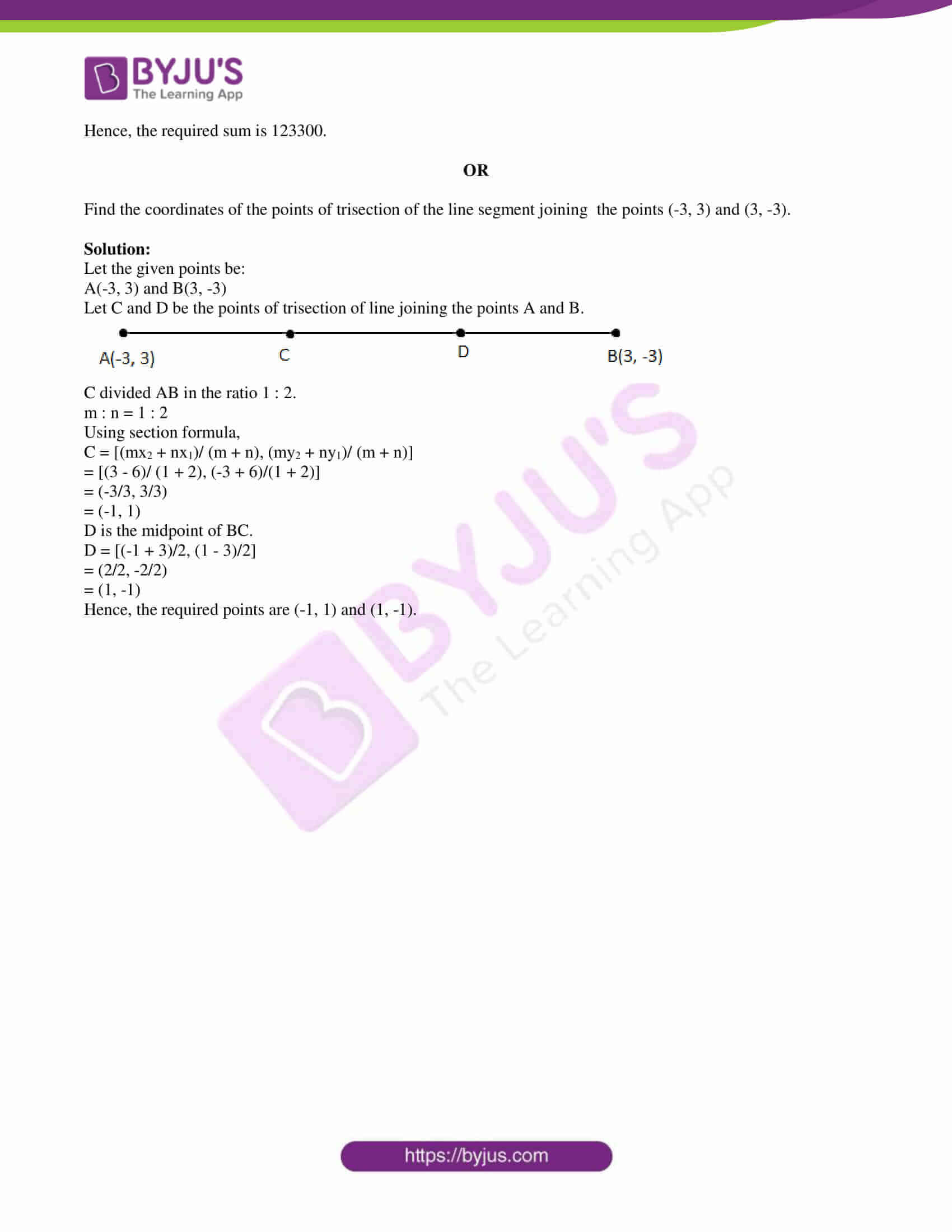PART A

SECTION – I

1. Find the value of log5 125.

Solution:

Let log5 125 = x

⇒ 5x = 125

⇒ 5x = 53

⇒ x = 3

Therefore, log5 125 = 3

2. If A = {1, 1/4, 1/9, 1/16, 1/25}, then write A in set-builder form.

Solution:

A = {1, 1/4, 1/9, 1/16, 1/25}

= {1/12, 1/22, 1/32, 1/42, 1/52}

The set-builder form: A = {1/x2 : x ≤ 5, x ∈ N}

3. Write an example for a quadratic polynomial that has no zeroes.

Solution:

x2 + x + 11 is one of the polynomials which do not have zeroes.

4. If b2 – 4ac > 0 in ax2 + bx + c = 0, then what can you say about roots of the equation? (a ≠ 0)

Solution:

Given,

ax2 + bx + c = 0

And

b2 – 4ac > 0

Hence, the roots of the equation are real and unequal.

5. Find the sum of the first 200 natural numbers.

Solution:

First 200 natural numbers: 1, 2, 3, 4,…,200

n = 200

We know that,

Sum of first n natural numbers = n(n + 1)/2

Sum of the first 200 natural numbers = 200(200 + 1)/2

= 100 × 201

= 20100

6. For what values of m, the pair of equations 3x + my = 10 and 9x + 12y = 30 have a unique solution.

Solution:

Given.

3x + my = 10

9x + 12y = 30

Comparing with the standard form a1x + b1y + c1 = 0 and a2x + b2y + c2 = 0,

a1 = 3, b1 = m, c1 = -10

a2 = 9, b2 = 12, c2 = -30

Condition for unique solution

a1/a2 ≠ b1/b2

3/9 ≠ m/12

⇒ m ≠ 12/3

⇒ m ≠ 4

Hence, m takes all the real values except 4.

7. Find the midpoint of the line segment joining the points (-5, 5) and (5, -5).

Solution:

Let the given points be:

(x1, y1) = (-5, 5)

(x2, y2) = (5, -5)

Midpoint = [(x1 + x2)/2, (y1 + y2)/2]

= [(-5 + 5)/2, (5 – 5)/2]

= (0/2, 0/2)

= (0, 0)

SECTION – II

8. If x2 + y2 = 7xy, then show that 2 log(x + y) = log x + log y + 2 log 3.

Solution:

Given,

x2 + y2 = 7xy

Adding 2xy on both the sides,

x2 + y2 + 2xy = 7xy + 2xy

(x + y)2 = 9xy

(x + y)2 = (3)2xy

Taking log on both sides,

log (x + y)2 = log (3)2xy

2 log (x + y) = log 32 + log x + log y

2 log (x + y) = 2 log 3 + log x + log y

9. Length of a rectangle is 5 units more than its breadth. Express its perimeter in polynomial form.

Solution:

Let x be the breadth of a rectangle.

Length = (x + 5) units

Perimeter of rectangle = 2 (Length + Breadth)

= 2(x + 5 + x)

= 2(2x + 5)

= 4x + 10

10. Measures of sides of a triangle are in Arithmetic Progression. Its perimeter is 30 cm and the difference between the longest and shortest side is 4 cm, then find the measures of the sides.

Solution:

Let a – d, a, a + d be the measures of three sides of a triangle.

According to the given,

Perimeter = 30 cm

a – d + a + a + d = 30

3a = 30

a = 30/3

a = 10

Also,

a + d – (a – d) = 4

2d = 4

d = 4/2

d = 2

Thus, a – d = 10 – 2 = 8

a + d = 10 + 2 = 12

Hence, the measures of the triangle are 8 cm, 10 am and 12 cm.

11. Show that the points A(-3, 3), B(0, 0), C(3, -3) are collinear.

Solution:

If (x1, y1), (x2, y2) and (x3, y3) are collinear, then the area of the triangle formed by these vertices is 0.

That means ½ [x1(y2 – y3) + x2(y3 – y1) + x3(y1 – y2)] = 0

Given,

A(-3, 3), B(0, 0), C(3, -3)

Area of triangle ABC = ½ [-3(0 + 3) + 0(-3 – 3) + 3(3 – 0)]

= ½ [-9 + 0 + 9]

= ½ (0)

= 0

Hence, the given points are collinear.

12. Solve the following pair of linear equations by substitution method.

2x – 3y = 19

3x – 2y = 21

Solution:

Given,

2x – 3y = 19….(i)

3x – 2y = 21….(ii)

From (i),

2x – 3y = 19

2x = 3y + 19

x = (3y + 19)/2….(iii)

Substituting (iii) in (ii),

3[(3y + 19)/2] – 2y = 21

9y + 57 – 4y = 42

5y = 42 – 57

5y = -15

y = -15/5

y = -3

Substituting y = -3 in (iii),

x = [3(-3) + 19)]/2

= (-9 + 19)/2

= 10/2

= 5

Hence, the solution of the given pair of linear equations is x = 5 and y = -3.

13. If 9x2 + kx + 1 = 0 has equal roots, find the value of k.

Solution:

Given,

9x2 + kx + 1 = 0

Comparing with the standard form ax2 + bx + c = 0,

a = 9, b = k, c = 1

Condition for equal roots:

b2 – 4ac = 0

k2 – 4(9)(1) = 0

k2 – 36 = 0

k2 = 36

k = √36

k = ±6

SECTION – III

14. Use Euclid’s division lemma to show that the cube of any positive integer is of the form 7m or 7m + 1 or 7m + 6.

Solution:

Let a be any positive integer and b = 7.

By Euclid’s division lemma,

a = bq + r, 0 ≤ r < b

a = 7q + r; r = 0, 1, 2, 3, 4, 5, 6

When r = 0,

a = 7q

a3 = (7q)3

a3 = 343q3

a3 = 7(49q3)

a3 = 7m, where m = 49q3

Also,in (7q + r)3, consider r3 and divide by 7. The remainder will give the result in each case.

When r = 1,

13 = 1 and a3 = 7m + 1

When r = 2,

23 = 8, divided by 7, the remainder is 1. Therefore, a3 = 7m + 1

When r = 3,

33 = 27 divided by 7, the remainder is 6. Therefore, a3 = 7m + 6

When r = 4,

43 = 64 divided by 7, the remainder is 1. Therefore, a3 = 7m + 1

When r = 5,

53 = 125 divided by 7, the remainder is 6. Therefore, a3 = 7m + 6

When r = 6,

63 = 216 divided by 7, the remainder is 6. Therefore, a3 = 7m + 6

Hence, the cube of any positive integer is of the form 7m or 7m + 1 or 7m + 6.

OR

Prove that √2 – 3√5 is an irrational number.

Solution:

Let √2 – 3√5 be a rational number.

√2 – 3√5 = a, where a is an integer.

Squaring on both sides,

(√2 – 3√5)2 = a2

(√2)2 + (3√5)2 – 2(√2)(3√5) = a2

2 + 45 – 6√10 = a2

47 – 6√10 = a2

-6√10 = a2 – 47

√10 = (47 – a2)/6

(47 – a2)/6 is a rational number since a is an integer.

Therefore, √10 is also an integer.

We know that integers are not rational numbers.

Thus, our assumption that √2 – 3√5 is a rational number is wrong.

Hence, √2 – 3√5 is an irrational number.

15. Draw the graph for the polynomial p(x) = x2 – 3x + 2 and find the zeroes from the graph.

Solution:

Given,

p(x) = x2 – 3x + 2

Let y = x2 – 3x + 2

 x -2 0 1 2 3 4 5 Y = p(x) 12 2 0 0 2 6 12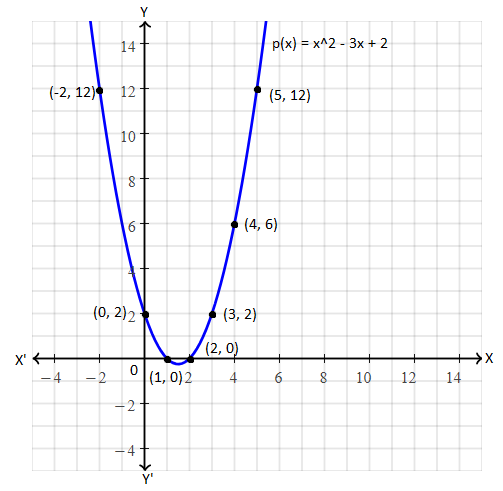OR

Draw the graph for the following pair of linear equations in two variables and find their solution from the graph.

3x – 2y = 2 and 2x + y = 6

Solution:

Given,

3x – 2y = 2

2x + y = 6

Consider the first equation:

3x – 2y = 2

2y = 3x – 2

y = (3/2)x – 1

 x -2 0 2 4 6 y -4 -1 2 5 8

Now, consider another equation:

2x + y = 6

y = -2x + 6

 x -2 0 2 3 4 y 10 6 2 0 -2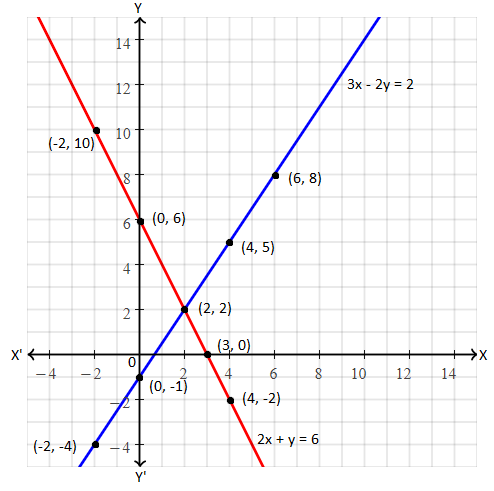The lines representing the given pair of equations intersecting each other at (2, 2).

Hence, the solution of the given pair of linear equations is x = 2 and y = 2.

16. Sum of the squares of two consecutive positive even integers is 100, find those numbers by using quadratic equations.

Solution:

Let x and x + 2 be the two consecutive even integers.

According to the given,

x2 + (x + 2)2 = 100

x2 + x2 + 4 + 4x – 100 = 0

2x2 + 4x – 96 = 0

2(x2 + 2x – 48) = 0

x2 + 2x – 48 = 0

x2 + 8x – 6x – 48 = 0

x(x + 8) – 6(x + 8) = 0

(x + 8)(x – 6) = 0

x = -8, x = 6

The value of x cannot be negative.

Thus, x = 6

x + 2 = 6 + 2 = 8

Hence, the required numbers are 6 and 8.

OR

X is a set of factors of 24 and Y is a set of factors of 36, then find sets X ⋃ Y and X ⋂ Y by using Venn diagram and comment on the answer.

Solution:

X = factors of 24

= {1, 2, 3, 4, 6, 8, 12, 24}

Y = factors of 36

= {1, 2, 3, 4, 6, 9, 12, 18, 36}

X ⋃ Y = {1, 2, 3, 4, 6, 8, 12, 24} ⋃ {1, 2, 3, 4, 6, 9, 12, 18, 36} = {1, 2, 3, 4, 6, 8, 9, 12, 18, 24, 36}

X ⋂ Y = {1, 2, 3, 4, 6, 8, 12, 24} ⋂ {1, 2, 3, 4, 6, 9, 12, 18, 36} = {1, 2, 3, 4, 6, 12}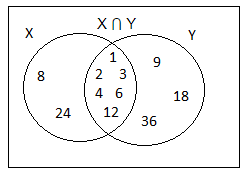17. Find the sum of all the three digit numbers, which are divisible by 4.

Solution:

Three digit numbers which are divisible by 4 are:

100, 104, 108,…., 996

This is an AP with a = 100 and d = 4

nth term of AP

an = a + (n – 1)d

996 = 100 + (n – 1)4

(n – 1)4 = 996 – 100 = 896

n – 1 = 896/4

n – 1 = 224

n = 225

Sum of first n terms

Sn = n/2(a + an)

S225 = (225/2) × (100 + 996)

= (225/2) × 1096

= 123300

Hence, the required sum is 123300.

OR

Find the coordinates of the points of trisection of the line segment joining the points (-3, 3) and (3, -3).

Solution:

Let the given points be:

A(-3, 3) and B(3, -3)

Let C and D be the points of trisection of line joining the points A and B.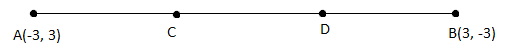C divided AB in the ratio 1 : 2.

m : n = 1 : 2

Using section formula,

C = [(mx2 + nx1)/ (m + n), (my2 + ny1)/ (m + n)]

= [(3 – 6)/ (1 + 2), (-3 + 6)/(1 + 2)]

= (-3/3, 3/3)

= (-1, 1)

D is the midpoint of BC.

D = [(-1 + 3)/2, (1 – 3)/2]

= (2/2, -2/2)

= (1, -1)

Hence, the required points are (-1, 1) and (1, -1).